UPSC  >  Important Formulas & Tips: Percentages

# Important Formulas & Tips: Percentages - CSAT Preparation - UPSC

## Percentages

The percent is derived from the Latin word ‘Centum’ which means for ‘every 100’ or ‘per hundredth.’

“Percentage is the representation of a number, ratio, fraction, or decimal as a fraction of 100.”

##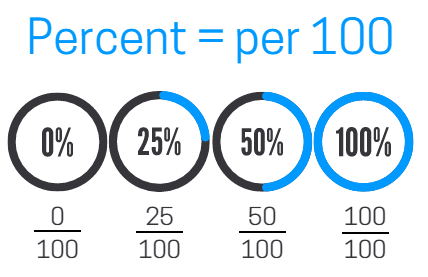Representing number, fraction, ratio and decimal in the form of Percentage

1. Number:  To represent a number ‘N’ into Percentage, just simply multiply the number with 100.

Example:  Convert ‘4’ into Percentage.
Sol: Representation of 4 in Percentage will be
= 4 × 100 = 400%

2. Fraction: To represent a fraction into Percentage, just simply multiply the fraction with 100 and to convert Percentage into fraction just simply divide the Percentage by 100.

Example: Convert the fraction 3/5 into Percentage
Sol: Representation of 3/5 into Percentage will be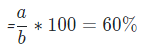3. Ratio: To convert the ratio into Percentage, first convert the ratio into a fraction and then multiply the fraction with 100.
Example: Convert the ratio 2 : 5 into Percentage?
Sol: Converting ratio into fraction we get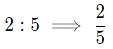Now, the representation of  2/5  into Percentage will be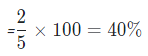4. Decimal: To represent a decimal into Percentage just simply multiply the decimal with 100 and to convert Percentage into decimal just simply divide the Percentage by 100.
Example: Convert 0.773 into percentage
Sol: Representation of 0.773 into percentage will be

=0.773 × 100=77.3%

To solve the questions based on Percentage, one should learn some important conversion of Percentages into Fractions.

Some other important fraction values in terms of Percentage are

NOTE: learning all the important fractions will save you a lot of Time.

• If there is 0.11, 022, ……., 0.88, and 0.99 in the Percentage, then the fraction corresponding to the Percentage will have 9 or multiple of 9 in the denominator.
• If there is 0.33 and 0.66 in the Percentage, then the fraction corresponding to the Percentage will have 3 or multiple of 3 in the denominator.
• If there is 0.1428, 0.2856, 0.4285, 0.5713, 0.7142 and 0.8571 in the Percentage, then the fraction corresponding to the Percentage will have 7 or multiple of 7 in the denominator.

### Increase/Decrease in Percentage

Percentage increase/decrease is the ratio of the change in number/quantity with respect to the original number/quantity.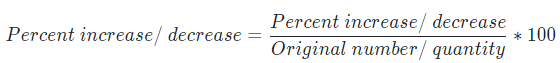• When A is increased by x%, then a new number B is obtained. To obtain the number A again, we have to decrease the number B by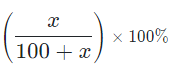• When A is decreased by y%, then a new number B is obtained. To obtain the number A again, we have to increase the number B by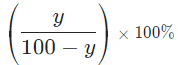Let us understand the above concept through questions

Example: Raghu has 20% more toffee than Pinki. How much percent less toffees Pinki have as compared to Raghu?
Sol:
Here, the quantity of toffees with Raghu is 20% more than Pinki, i.e., the value of x is 20%

So, Percentage of toffees with Pinki as compared to Raghu will be less by: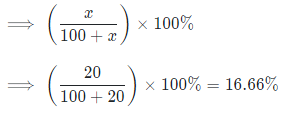Note: When ‘percentage increased/decreased by X%’ is given for a quantity, then the actual increase/decrease in the quantity is (100± X%)

While when ‘percentage increase/decrease to X%’ is given for a quantity, then the actual increase/decrease in the quantity is (±X%)

For example, if the salary of a person is decreased by 20%, then the final salary of the person will be 80% of the original salary while if the salary of a person is decreased to 20% of the final salary of the person will be 20% of the original salary.

Concept of Product constancy

Product constancy involves the problems with two dependent quantities whose product is the third quantity. Thus, if there is an increase in the first quantity, then to make the third quantity constant, we have to increase/decrease the second quantity.

Some relations from which questions on product constancy are asked are:

• Expenditure = Price * Consumption
• Price = Rate * Quantity
• Distance = Speed * Time
• Work done = Time taken * Efficiency
• Area of Rectangle = Length * Breadth

Example: The price of sugar is increased by 25%. If a family does not want to increase/decrease their expenditure, then by what percent should it decrease their consumption?
Sol: We know, Expenditure = Price * Consumption
As, price is increased by 25%
So, here x = 25%
Thus, to remain the expenditure constant we should decrease the consumption by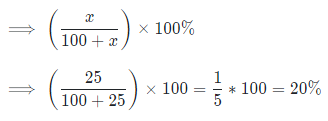Quantities having relation in the form of C = A + B

In three quantities having a relation of C = A + B, quantity A is increased by x%. If we wish to maintain C as constant, then the B should be reduced by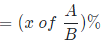and vice versa in case of a decrease.

Example: The saving of a person is 20% of his income. If his expenditure increases by 10% without any increase in his income, then by how much percent he has to decrease his saving?
Sol:
Let us assume income of the person be 100

So, initial savings of the person = 20% of 100 = 20

We know, Income = Expenditure + Saving

Expenditure of person = 100 – 20 = 80

According to the question,

Final expenditure of person = 110% of 80 = 88

As, income is constant so final income = 100

Final Saving = 100 – 88 = 12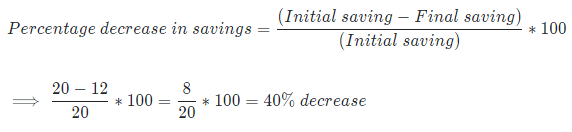### Successive Percentage increase/decrease

If a number N undergoes a successive percentage increase/decrease of a%, b%, c% and so on then after a net change in the number, the final value of number will be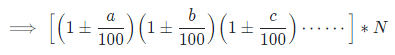Application of Percentage on Population increase/decrease

If there is an r% increase/decrease in population of a place in a year, then

The Population of a place n years later will be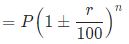And, population of a place n years ago will be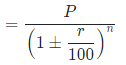## Practice Questions

Q1. If 5/9  is multiplied instead of  2/3 in a number, then what will be the percentage error in the calculation?
Sol: Let the number be LCM of 9 and 3 = 9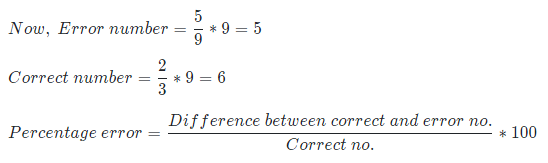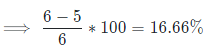Q2. In an examination, out of 400 students, 54% of boys and 70% of girls pass. If the total pass percentage was 60%. Find the total number of girls.

Sol: Let the number of girls be x
Thus, number of boys will be 400 – x
According to the question
Number of boys who pass = 54% of (400 – x)
Number of girls who pass = 70% of x
Total number of students who pass = 60% of 400
Thus,
60% of 400 = 54% of (400 – x) + 70% of x
240 = 216 – 0.54x + 07x
0.16x = 24
x = 150

Q3. The population of a town increases by 20% each year. If the population of the town 3 years ago was 2500, then what is the present population of the town?
Sol: Let us assume the present population of the town is P

We know,

The population of a place n years ago will be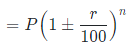According to the question,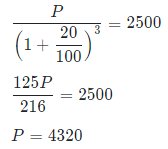Thus, the present population of the town is 4320.

Q4. If A earns 20% more than B, B earns 25% more than C, C earns 16.67% less than D, then A earns how much percent of D?
Sol: We know,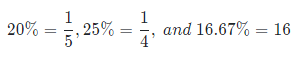According to the question,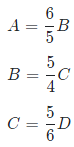Combining all the above equation,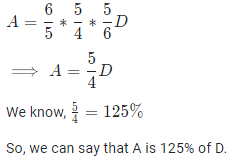The document Important Formulas & Tips: Percentages | CSAT Preparation - UPSC is a part of the UPSC Course CSAT Preparation.
All you need of UPSC at this link: UPSC

## CSAT Preparation

197 videos|151 docs|200 tests

## FAQs on Important Formulas & Tips: Percentages - CSAT Preparation - UPSC

 1. What are some basic formulas for percentages?Ans. Some basic formulas for percentages are: - Percentage = (Part/Whole) x 100 - Part = (Percentage/100) x Whole - Whole = (Part/Percentage) x 100 These formulas can be used to find any one of the three values given the other two.
 2. How do I calculate percentage increase or decrease?Ans. To calculate percentage increase or decrease, you can use the following formula: Percentage change = ((New value - Old value)/Old value) x 100 If the result is positive, it represents a percentage increase. If the result is negative, it represents a percentage decrease.
 3. How do I find the percentage of a number?Ans. To find the percentage of a number, you can use the following formula: Percentage = (Part/Whole) x 100 For example, if you want to find 20% of 50, you would use the formula: Percentage = (20/100) x 50 = 10 So 20% of 50 is 10.
 4. How can I use percentages to make comparisons?Ans. Percentages can be used to make comparisons between two or more values. For example, if you want to compare the sales of two products, you can calculate the percentage difference in sales between the two products. To do this, you can use the following formula: Percentage difference = ((Value 1 - Value 2)/((Value 1 + Value 2)/2)) x 100 The result will give you the percentage difference between the two values. A positive result means that the first value is larger, while a negative result means that the second value is larger.
 5. How do I convert a decimal to a percentage?Ans. To convert a decimal to a percentage, you can multiply the decimal by 100 and add a percentage sign. For example, if you have a decimal of 0.75, you can convert it to a percentage by multiplying it by 100: 0.75 x 100 = 75% So 0.75 is equal to 75%.

## CSAT Preparation

197 videos|151 docs|200 testsExplore Courses for UPSC exam### How to Prepare for UPSC

Read our guide to prepare for UPSC which is created by Toppers & the best Teachers
Signup to see your scores go up within 7 days! Learn & Practice with 1000+ FREE Notes, Videos & Tests.
10M+ students study on EduRev
Track your progress, build streaks, highlight & save important lessons and more!
Related Searches

,

,

,

,

,

,

,

,

,

,

,

,

,

,

,

,

,

,

,

,

,

;